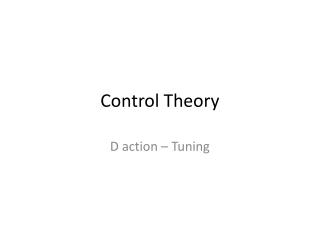DownloadDownload PresentationControl Theory

# Control Theory

Télécharger la présentation## Control Theory

- - - - - - - - - - - - - - - - - - - - - - - - - - - E N D - - - - - - - - - - - - - - - - - - - - - - - - - - -
##### Presentation Transcript

1. ControlTheory D action – Tuning

2. Derivative Action • Whenthere’stoomuchoscillation, thiscansometimesbesolvedbyadding a derivative action. Thisaction willtake into account howfast the error signal is changing. • In the time domain this leads to: • the PD controller: • and the PID controller:

3. Derivative Action PD in the s domain: PID in the s domain:

4. Group Task r(t) z(t) 2/(s2+2s) P or PD? Controller Process Compare a P controller withKc=10 with a PD controller withKc=10 and TD=0,2 (step response for the servoproblem)

6. Tuning Tuning = choosing appropriate parameters for the controller For PID control: choice of Kc, TI and TD • 2 possibilities: • Based on (open/closed loop) model • Based on experiments • 2 prettymuchusedmethods: • Ziegler-Nichols • Cohen-Coon (notdiscussed)

7. Tuning • (one type of) ZIEGLER-NICHOLS: • Start with a P controllerwith low Kcandincreasethisgainslowlyuntilyoscillateswith a constant amplitude. • (Remark: every time Kc is increased, oneshouldbring the closed loop system out of equilibrium, e.g. byslightlychanging the reference!) • 2) The gain we have now is called the ultimate gainKuand the period of the oscillation is called the ultimate periodTu • 3) Choose the PID parameters accordingto the followingtable:

8. Tuning Example: A process with the following step response:

9. Tuning Example: Ku = 1.45 Tu = 4.5s bv. PID: Kc = 0.87 TI = 2.25s TD = 0.65s

10. Tuning Example: Ku = 1.45 Tu = 4.5s bv. PID: Kc = 0.87 TI = 2.25s TD = 0.65s 1 5 10 15 Remark: ZN typically leads to pretty much overshoot... (there are revised ZN methods that solve this problem)

11. Tuning Example: Suppose a model of the process was given by Can you now tune according to ZN based on this model?

13. Problem(s) introduced by D-control? • Control action in servo problem becomes “infinitely” high. • High frequencybehavior/noise is amplified. • None of the above. • Both A and B [Default] [MC Any] [MC All]

14. Bode plots! Bode plot PD:

15. Derivative Action with filtering We introduce an extra pole to solve the problem at high frequencies: This means we first filter the error with a low pass filter. Off course the time constant of the filtering << TD (Remark: to solve the problem of suddenly changing reference values, often another solution is presented: the derivative action is put on the MEASUREMENT instead of on the ERROR!)

16. Derivative Action with filtering Difference in Bode plot: Blue: D action Green: D action + prefiltering Remark: The positive effect on the phase shift is now lost at higher frequencies!

17. Influence of a time delay 1) What is a time delay? Typical example: measurement comes too late, e.g.: TR TR TR,m TR,m td

18. Influence of a time delay In other words: f(t-td) f(t) Dead time td This is of course a pure phase shift. In Laplace (see table): e-td s F(s) F(s) Dead time td A dead time is given as e-td s in the s domain: it’snon-linear! On the Bodeplot? AR = |e-tdjω|=1 φ = (e-tdjω) = -tdω (in rad) Pure phase shift

19. CHAPTER 3. PID CONTROL Influence of dead time 2) What is the influence on a feedback system? A- Bode plot of a time delay:

20. Influence of a time delay 2) What is the influence on a feedback system? φ = (e-tdjω) = -tdω * 180/π (in degrees) • Thiscan have severe impact on the stability: • The information comestoo late. • How can we seethis in our analysis? • Based on TF: difficult: time delay = non-linearthing • … but it is a pure phase shift… on Bode plot?